# Backstepping Direct Power Control for Power Quality Enhancement of Grid-connected Photovoltaic System Implemented with PIL Co-simulation Technique

Backstepping Direct Power Control for Power Quality Enhancement of Grid-connected Photovoltaic System Implemented with PIL Co-simulation Technique

Brahim Elkhalil YoucefaAhmed Massoum Said Barkat Patrice Wira

Département d’Electrotechnique, Faculté des Sciences de l’Ingénieur, Université Djillali Liabes de Sidi Bel Abbes, Sidi Bel Abbés 22000, Algérie

Laboratoire de Génie Electrique, Université de M’sila, M’sila 28000, Algérie

Laboratoire lRlMAS, Université de Haute Alsace, Mulhouse 68093, France

Corresponding Author Email:
khalilyoucefa@gmail.com
Page:
1-14
|
DOI:
https://doi.org/10.18280/ama_c.740101
1 November 2018
| |
Accepted:
16 February 2019
| | Citation

OPEN ACCESS

Abstract:

This paper proposes a combined nonlinear backstepping approach with direct power control technique for improving power quality of a three-phase grid-connected solar energy conversion system. The presented system basically extracts maximum power from solar photovoltaic array, converts it into AC power via a voltage source converter, and supplies it to the grid and connected loads. The proposed system offers not only the function of grid connected PV system but also it acts as a shunt active power filter (PV-SAPF). The system intends to eliminate the poor power quality issues and provides current conditioning while operating in coherence under nonlinear load variations. In order to validate the proposed double function system, processor-in-the-loop (PIL) tests are carried out for steady state and dynamic regimes under a nonlinear load operating condition.

Keywords:

grid connected PV system, shunt active filter, backstepping control, direct power control, power quality, processor-in-the-loop

1. Introduction

Photovoltaic (PV) technology is becoming one of the foremost alternative solutions to produce electrical energy owing to the broad sustainability of solar irradiances. In fact, PV technology offers many advantages such as clean, renewable, noiseless, and ease of implementation. However, this technology is characterized by low electrical energy generation; its applications are limited to low power systems for instance watches, LED lighting, and some standalone electrical systems. For its application at medium and high power levels, like grid-connected systems, the PV energy must be adapted using appropriate power converters. Thanks to these interfacing electronic systems, the PV source can be fully operational by forcing it to deliver a maximum of energy to the utility grid .

It is well established that, the increased demand for nonlinear loads and renewable energy resources influence the power networks performance from the perspective of power quality . Consequently, the integration of grid-connected photovoltaic system in distribution systems supplying nonlinear loads is not only unable to alleviate the power fluctuation, but it may make the situation worse depending on the PV inverter technology . The most meaningful solution to these issues is the resort to power filters. However, the use of passive filter or separate active filter in grid-connected system raises its size, weight, and cost, which are the main shortfalls among others of this solution. To surmount these defects, PV system itself acting as a shunt active power filter has been proposed by multitude researches as indicated in the literature [4-7]. Indeed, by injecting the appropriate compensating current into the grid, the filtering function of the PV system can improve the current harmonic distortion, power conversion efficiency, and reliability [8-9].

In order to improve the performances of the PV system acting as a shunt active power filter, several nonlinear control methods were reported in the literature, which go beyond the limitations of the linear controllers . Feedback linearization technique used to improve the control of the APF is presented in . Another control strategy based on Lyapunov stability theory is studied in  for single-phase shunt active power filters. In , a nonlinear control technique for a three-phase SAPF is proposed and tested in a laboratory prototype. A robust control for the shunt active filter using fuzzy logic controller was studied in . A nonlinear control based on feedback linearization technique for a single-phase SAPF is presented in . On the other hand, adaptive sliding mode control, studied in , is designed for a boost converter with an unknown resistive load and external input voltage. Feedback linearization control strategy is widely used for a cascade nonlinear control of DC-DC boost converter to provide satisfactory performances over a wide range of operating points .

Over the last few years, direct power control (DPC) has become more widely used when compared with other methods [18-19] because of its advantages like fast dynamic performance and simple control implementation. This method coming from the popular direct torque control (DTC)  that is applied in electrical machines control. Nonetheless, In DPC method, neither the internal current control loops nor the PWM modulator bloc are needed, for the simple raison that the inverter switching states are selected by a switching table founded on the instantaneous power errors and voltage vector position. However, the variation of switching frequency is the main disadvantage of DPC , which generates an unwanted spectrum range of broadband harmonic and makes it hard to design a line filter . By using space vector modulation (SVM) algorithm that replaces the conventional switching table, these disadvantages can be efficiently overcome. The combination of DPC and SVM forms the so-called space vector modulation direct power control (SVM-DPC) .

In this paper, a nonlinear backstepping control method combined with direct power control is proposed to control a photovoltaic system acting as a shunt active power filter. The main tasks of the filtering system are harmonic currents reduction and reactive power compensation. Ideally, the presented system needs to generate enough reactive power and harmonic currents to compensate for the harmful effect of nonlinear loads on the grid. Moreover, to extract maximum amount of power from the photovoltaic generator, a suitable backstepping current control method for the DC-DC boost converter is also developed.

The rapid development of the computer system and user interactive software allows a lot of simulation software, which are available for technical applications. In this paper, all system control methods using simulation block sets are formed and transformed into embedded C code. The generated code is launched into an embedded processor; this test is called the processor-in-the-loop (PIL). In addition, this approach standardized the designer with a program and reduced the core technical labor force effort, in the same time gives more functionality to test the control methods for a system in a real DSP board. The software MATLAB/Simulink is used as simulation platform, and STM32F429i-Discovery board is used as a target to launch the program in a processor.

This paper is organized as follows: in section II, description and modeling of the SAPF side system are given. In section III, synthesis and design of the proposed controllers for the SAPF are developed. In section IV, the control synthesis of the DC-DC boost converter is presented. In section V, processor-in-the-loop is described, and co-simulation results are given and discussed. Finally, the paper is concluded in its last section.

2. System Description and Modeling

2.1 System description

The utility grid is supposed to be a sinusoidal voltage source with series short circuit impedances. The grid is modeled by three-phase electromotive forces in series with impedances as shown in Figure. 1. In the right side of the system, a nonlinear load is connected to the utility grid through intermediate line impedances (Ll,Rl). This load is composed of uncontrolled three-phase rectifier supplying a load (Rd, Ld) in its DC side. In the same Figure, a DC-DC boost converter is utilized to interface the photovoltaic generator with the grid across a voltage source inverter (VSI). The inverter, connected in parallel at the point of common coupling (PCC), acts, in the same time, as a PV inverter and SAPF where it is often controlled as a current generator. For the purpose to make grid current pure sinusoidal, the active filter injects unbalanced currents equal and in phase opposition of those absorbed by the nonlinear load.

Briefly, the active filter function of the proposed system prohibits disturbance currents generated by the nonlinear load to circulate through the grid impedances. Thus, the resulting total currents drawn from the AC main are pure sinusoidal and balanced.

## 1.pngFigure 1. Power circuit of grid-connected photovoltaic system acting as a shunt active power filter

## 2.pngFigure 2. Block diagram of the proposed backstepping direct power control method for the PV-SAPF

2.2 Mathematical model of the SAPF

The system equations defining the SAPF in the three-phase reference frame are given by:

${ L } _ { { f } } \frac { { d } { i } _ { { fa } } } { { dt } } = - { R } _ { { f } } { i } _ { { fa } } + { v } _ { { fa } } - { V } _ { { a } }$

${ L } _ { { f } } \frac { { d } { i } _ { { fb } } } { { dt } } = - { R } _ { { f } } { i } _ { { fb } } + { v } _ { { fb } } - { V } _ { { b } }$

${ L } _ { { f } } \frac { { d } { i } _ { { fc} } } { { dt } } = - { R } _ { { f } } { i } _ { { fc } } + { v } _ { { fc } } - { V } _ { { c } }$

${ C } _ { { dc } } \frac { { d } { V } _ { { dc } } } { { dt } } = - { S } _ { { a } } { i } _ { { fa } } + { S } _ b i_{ fb } + S_c { i } _ { { fc } }$      (1)

where ifi, vfi with i=a,b,c represent the AC side currents and voltages of the SAPF, respectively; vi, i=a,b,c are the point of common coupling voltages; Lf, Rf are the output inductance and resistance of the shunt active power filter, respectively; Si, i=a,b,c are the control signals for the VSI,  Vdc is the voltage across the DC capacitor Cdc.

The mathematical model of SAPF in the stationary reference is given as follows:

${ L } _ { { f } } \frac { { d } { i } _ { { f\alpha } } } { { dt } } = - { R } _ { { f } } { i } _ { { f\alpha } } + { v } _ { { f\alpha } } - { V } _ { { \alpha } }$

${ L } _ { { f } } \frac { { d } { i } _ { { f\beta } } } { { dt } } = - { R } _ { { f } } { i } _ { { f\beta } } + { v } _ { { f\beta } } - { V } _ { { \beta } }$

${ C } _ { { dc } } \frac { { d } { V } _ { { dc } } } { { dt } } = \frac{P_{dc}}{V_{dc}} (2) where if, vfi with i=α,β represent the AC side current and voltage components of the SAPF in the stationary reference, respectively, vα, vβ are the point of common coupling voltages in the stationary reference, Pdc is the DC active power across the capacitor Cdc. 2.3 Mathematical model of the SAPF for powers control The powers at the output of the SAPF are given as:$\left[ \begin{array} { c } { \mathrm { P } _ { \mathrm { F } } } \\ { \mathrm { Q } _ { \mathrm { F } } } \end{array} \right] = \left[ \begin{array} { c c } { \mathrm { V } _ { \alpha } } & { \mathrm { V } _ { \beta } } \\ { - \mathrm { V } _ { \beta } } & { \mathrm { V } _ { \alpha } } \end{array} \right] \left[ \begin{array} { c } { \mathrm { i } _ { \mathrm { f } \alpha } } \\ { \mathrm { i } _ { \mathrm { f } \beta } } \end{array} \right]$(3) In order to calculate the powers derivatives, the Lie derivative method is used ; the active and reactive powers must be chosen as outputs. The two first equations of the system (2) can be written as follow:$\frac { \mathrm { d } \mathrm { x } } { \mathrm { dt } } = \mathrm { f } ( \mathrm { x } ) + \mathrm { g } ( \mathrm { x } ) \mathrm { u }\mathrm { y } = \mathrm { h } ( \mathrm { x } )$(4) where$x = \left[ \begin{array} { c } { i _ { f \alpha } } \\ { i _ { f \beta } } \end{array} \right] , f ( x ) = \left[ \begin{array} { c } { f _ { 1 } } \\ { f _ { 2 } } \end{array} \right] = \frac { 1 } { L _ { f } } \left[ \begin{array} { c } { - R _ { f } i _ { f \alpha } - v _ { \alpha } } \\ { - R _ { f } i _ { f \beta } - v _ { \beta } } \end{array} \right]g ( x ) = \frac { 1 } { L _ { f } } \left[ \begin{array} { l l } { 1 } & { 0 } \\ { 0 } & { 1 } \end{array} \right] , u = \left[ \begin{array} { l } { v _ { f \alpha } } \\ { v _ { f \beta } } \end{array} \right] , y = \left[ \begin{array} { l } { h _ { 1 } } \\ { h _ { 2 } } \end{array} \right] = \left[ \begin{array} { l } { P _ { F } } \\ { Q _ { F } } \end{array} \right]$where f(x) and h(x) are a second order smooth vector fields, g(x) is an 2x2 matrix of smooth vector field columns, u and y are 2x1 vectors of input and output respectively. The derivative of each output can be expressed as follows :$\frac { \mathrm { dy } _ { \mathrm { i } } } { \mathrm { dx } } = \mathrm { L } _ { \mathrm { i } } \mathrm { h } _ { \mathrm { i } } + \mathrm { L } _ { \mathrm { g } } \mathrm { h } _ { \mathrm { i } } \mathrm { u }$(5) where$\mathrm { L } _ { \mathrm { i } } \mathrm { h } _ { \mathrm { i } }$,$\mathrm { L } _ { \mathrm { g } } \mathrm { h } _ { \mathrm { i } }$are the Lie derivatives of hi with respect to f and g, respectively. The derivatives of powers are given as:$\frac { \mathrm { d } \mathrm { P } _ { \mathrm { F } } } { \mathrm { dt } } = \mathrm { v } _ { \alpha } \mathrm { f } _ { 1 } + \mathrm { v } _ { \beta } \mathrm { f } _ { 2 } + \frac { \mathrm { V } _ { \alpha } } { \mathrm { L } _ { \mathrm { f } } } \mathrm { v } _ { \mathrm { f } \alpha } + \frac { \mathrm { V } _ { \beta } } { \mathrm { L } _ { \mathrm { f } } } \mathrm { V } _ { \mathrm { f } \beta }\frac { \mathrm { d } \mathrm { Q } _ { \mathrm { F } } } { \mathrm { dt } } = - \mathrm { v } _ { \beta } \mathrm { f } _ { 1 } + \mathrm { v } _ { \alpha } \mathrm { f } _ { 2 } - \frac { \mathrm { V } _ { \beta } } { \mathrm { L } _ { \mathrm { f } } } \mathrm { V } _ { \mathrm { f } \alpha } + \frac { \mathrm { V } _ { \alpha } } { \mathrm { L } _ { \mathrm { f } } } \mathrm { V } _ { \mathrm { f } \beta }$(6) After more simplification, the final new model of the SAPF is given as follows:$\frac { \mathrm { dP } _ { \mathrm { F } } } { \mathrm { dt } } = \frac { 1 } { \mathrm { L } _ { \mathrm { f } } } \left( - \mathrm { R } _ { \mathrm { f } } \mathrm { P } _ { \mathrm { F } } + \delta _ { \mathrm { f } \alpha } \right)\frac { \mathrm { dQ } _ { \mathrm { F } } } { \mathrm { dt } } = \frac { 1 } { \mathrm { L } _ { \mathrm { f } } } \left( - \mathrm { R } _ { \mathrm { f } } \mathrm { Q } _ { \mathrm { F } } + \delta _ { \mathrm { f } \beta } \right)\frac { dV  _ { dc} } { dt } = \frac { P_{dc}} { V_{dc}C_{dc}}$(7) where$\delta _ { f \alpha } = v _ { \alpha } v _ { f \alpha } + v _ { \beta } v _ { f \beta } - \left( v _ { \alpha } ^ { 2 } + v _ { \beta } ^ { 2 } \right)\delta _ { f \beta } = - v _ { \beta } v _ { f \alpha } + v _ { \alpha } v _ { f \beta }$3. Control Strategy for the Sapf Side In Figure. 2, the actual capacitor square voltage$V^2_{dc}$is compared with its reference square value$V^{*2}_{dc}$; the error between the capacitor voltage and its reference is fed to a nonlinear controller. The output of the nonlinear voltage controller presents the active power reference$P^*_{dc}$across the capacitor Cdc. Based on the instantaneous p–q theory, the compensating powers are calculated, and theaverage powers are extracted using 4-order low-pass filter (LPF). However, the oscillating powers are obtained through a simple subtraction of the average power from the active and reactive powers. 3.1 p–q theory based control strategy The instantaneous active and reactive powers of the nonlinear load are calculated as follows:$\left[ \begin{array} { c } { \mathrm { P } _ { \mathrm { L } } } \\ { \mathrm { Q } _ { \mathrm { L } } } \end{array} \right] = \left[ \begin{array} { c c } { \mathrm { v } _ { \alpha } } & { \mathrm { V } _ { \beta } } \\ { - \mathrm { V } _ { \beta } } & { \mathrm { V } _ { \alpha } } \end{array} \right] \left[ \begin{array} { l } { \mathrm { i } _ { \mathrm { L } \alpha } } \\ { \mathrm { i } _ { \mathrm { L } \beta } } \end{array} \right]$(8) The instantaneous active and reactive powers, including average and oscillating values, are expressed as follows:$\begin{aligned} \mathrm { P } _ { \mathrm { L } } & = \overline { \mathrm { P } } _ { \mathrm { L } } + \tilde { \mathrm { P } } _ { \mathrm { L } } \\ \mathrm { Q } _ { \mathrm { L } } & = \overline { \mathrm { Q } } _ { \mathrm { L } } + \tilde { \mathrm { Q } } _ { \mathrm { L } } \end{aligned}$(9) The average values ($\overline{P}_L,\overline{Q}_L$) of${P}_L$and${Q}_L$are the average active and reactive powers originating from the positive-sequence component of the nonlinear load current. Oscillating values ($\tilde{P}_L,\tilde{Q}_L$) of${P}_L$and${Q}_L$are the ripple active and reactive powers . The filter active and reactive power references are calculated as follows:$\mathrm { P } _ { \mathrm { F } } ^ { * } = \widetilde { \mathrm { P } } _ { \mathrm { L } } - \mathrm { P } _ { \mathrm { dc } } ^ { * } + \mathrm { P } _ { \mathrm { PV } }\mathrm { Q } _ { \mathrm { F } } ^ { * } = \mathrm { Q } _ { \mathrm { L } }$(10) The power reference of the DC-link capacitor${P}^*_{dc}$is used as an average real power and is obtained from the nonlinear backstepping DC voltage controller, while the${P}_{PV}$is the active power delivered from the photovoltaic generator used as a compensating power, (${P}_F$,${Q}_F$) are the active and reactive filter powers, respectively. 3.2 SAPF backstepping controllers design The backstepping algorithm is based on the idea that specific variables can be utilized as virtual controls to make the original high order system simple. So, the final control outputs can be established step by step through suitable Lyapunov functions that guarantee the global stability . On the contrary of other methods, the backstepping control method does not have constraints on the type of non-linearities, all control objectives are in fact achieved by using tools from the Lyapunov stability. This control method was successfully applied on a growing collection of plants [25-27]. In the following, the backstepping control strategy will be used to design SAPF controllers. The relied backstepping controllers are determined based on a decomposition of the global SAPF model. In order to accomplish this task, the system (7) is subdivided into three subsystems, as follows: Subsystem 1:$\frac { \mathrm { d } \mathrm { V } _ { \mathrm { dc } } } { \mathrm { dt } } = \frac { \mathrm { P } _ { \mathrm { dc } } } { \mathrm { V } _ { \mathrm { dc } } \mathrm { C } _ { \mathrm { dc } } }$(11) In the first subsystem described by the equation (11), the instantaneous active power$P^*_{dc}$is considered as a control variable and the voltage Vdc is considered as an output variable. Subsystem 2: In the second subsystem given by the equation (12), the voltage${\delta}^*_{f\alpha}$is chosen as a variable control, while the power PF as an output variable.$\frac { \mathrm { d } \mathrm { P } _ { \mathrm { F } } } { \mathrm { dt } } = \frac { 1 } { \mathrm { L } _ { \mathrm { f } } } \left( - \mathrm { R } _ { \mathrm { f } } \mathrm { P } _ { \mathrm { F } } + \delta _ { \mathrm { fa } } ^ { * } \right)$(12) Subsystem 3: In this subsystem,${\delta}^*_{f\beta}$and QF are the control variable and the output variable, respectively.$\frac { \mathrm { d } \mathrm { Q } _ { \mathrm { F } } } { \mathrm { dt } } = \frac { 1 } { \mathrm { L } _ { \mathrm { f } } } \left( - \mathrm { R } _ { \mathrm { f } } \mathrm { Q } _ { \mathrm { F } } + \delta _ { \mathrm { f } \beta } ^ { * } \right)$(13) 3.2.1 DC-link voltage backstepping controller synthesis To preserve the DC-link voltage across the capacitor Cdc at a constant desired value, a backstepping controller for DC-link voltage is used to maintain the DC-link voltage at its reference value covering the inverter losses. Since the purpose of this control is to force the DC-link voltage to follow its reference, the tracking variable error z1 is defined by:$\mathrm { z } _ { 1 } = \mathrm { V } _ { \mathrm { dc } } ^ { * } - \mathrm { V } _ { \mathrm { dc } }$(14) Using the first subsystem, the dynamics of the error z1 is given by:$\frac { \mathrm { d } \mathrm { z } _ { 1 } } { \mathrm { dt } } = \frac { \mathrm { d } \mathrm { V } _ { \mathrm { dc } } ^ { * } } { \mathrm { dt } } - \frac { \mathrm { P } _ { \mathrm { dc } } ^ { * } } { \mathrm { V } _ { \mathrm { dc } } \mathrm { C } _ { \mathrm { dc } } }$(15) The Lyapunov candidate function is chosen as:$\mathrm { v } _ { 1 } = \frac { 1 } { 2 } \mathrm { z } _ { 1 } ^ { 2 }$(16) The derivative of the function (16) is expressed as:$\frac { \mathrm { d } \mathrm { V } _ { 1 } } { \mathrm { dt } } = \mathrm { z } _ { 1 } \left( \frac { \mathrm { d } \mathrm { V } _ { \mathrm { dc } } ^ { * } } { \mathrm { dt } } - \frac { \mathrm { P } _ { \mathrm { dc } } ^ { * } } { \mathrm { V } _ { \mathrm { dc } } \mathrm { C } _ { \mathrm { dc } } } \right)$(17) To ensure the stability of the system, the derivative of the Lyapunov function must be negative. This can be established by choosing the derivative of z1 as:$\frac { \mathrm { d } \mathrm { z } _ { 1 } } { \mathrm { dt } } = - \mathrm { k } _ { 1 } \mathrm { z } _ { 1 }$(18) where k1 is a positive gain. Hence, the control law can be given by the equation (19) below and its controller block diagram is presented in Figure. 3.$\mathrm { P } _ { \mathrm { dc } } ^ { * } = \mathrm { V } _ { \mathrm { dc } } \mathrm { C } _ { \mathrm { dc } } \left( \frac { \mathrm { d } \mathrm { V } _ { \mathrm { dc } } ^ { * } } { \mathrm { dt } } + \mathrm { k } _ { 1 } \mathrm { z } _ { 1 } \right)$(19) ## 3.png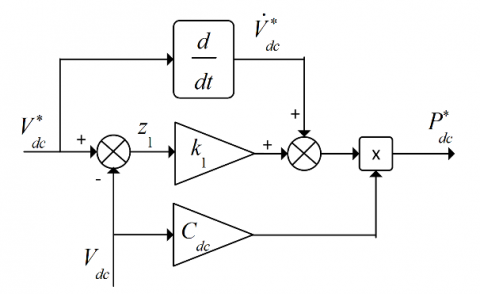Figure 3. Controller block diagram of the DC-link voltage backstepping controller 3.2.2 Active power backstepping controller synthesis The synthesis of a desired backstepping regulator for the active power PF using the second subsystem defined by the equation (12) is analyzed as follows: The variable error z2 is defined by:$z_2=P^*_F-P_F$(20) The dynamic of the error z2 is given by:$\frac { \mathrm { d } \mathrm { z } _ { 2 } } { \mathrm { dt } } = \frac { \mathrm { d } \mathrm { P } _ { \mathrm { F } } ^ { * } } { \mathrm { dt } } - \frac { 1 } { \mathrm { L } _ { \mathrm { f } } } \left( - \mathrm { R } _ { \mathrm { f } } \mathrm { P } _ { \mathrm { F } } + \delta _ { \mathrm { fa } } ^ { * } \right)$(21) The Lyapunov candidate function is chosen as:$V_2=\frac{1}{2}z^2_2$(22) The derivative of the function (22) is:$\frac { \mathrm { d } \mathrm { V } _ { 2 } } { \mathrm { dt } } = \mathrm { z } _ { 2 } \left( \frac { \mathrm { d } \mathrm { P } _ { \mathrm { F } } ^ { * } } { \mathrm { dt } } - \frac { 1 } { \mathrm { L } _ { \mathrm { f } } } \left( - \mathrm { R } _ { \mathrm { f } } \mathrm { P } _ { \mathrm { F } } + \delta _ { \mathrm { f } \alpha } ^ { * } \right) \right)$(23) To ensure the stability of the system, the derivative of the Lyapunov function must be negative; this can be accomplished by choosing the derivative of z2 as:$\frac { \mathrm { d } \mathrm { z } _ { 2 } } { \mathrm { dt } } =-k_2z_2$(24) with k2 is a positive gain. So, the obtained control law is given by the equation (25) while the Figure. 4 presents its controller block diagram.$\delta _ { \mathrm { fa } } ^ { * } = \mathrm { R } _ { \mathrm { f } } \mathrm { P } _ { \mathrm { F } } + \mathrm { L } _ { \mathrm { f } } \mathrm { k } _ { 2 } \mathrm { z } _ { 2 } + \mathrm { L } _ { \mathrm { f } } \frac { \mathrm { d } \mathrm { P } _ { \mathrm { F } } ^ { * } } { \mathrm { dt } }$(25) ## 4.pngFigure 4. Backstepping controller block diagram of the active power Once the intermediate voltage${\delta}^*_{f\alpha}$is obtained, the reference voltage$v^*_{f\alpha}$can be calculated using (7) as follows:$\mathrm { v } _ { \mathrm { f } \alpha } ^ { * } = \frac { \mathrm { v } _ { \alpha } } { \mathrm { v } _ { \alpha } ^ { 2 } + \mathrm { v } _ { \beta } ^ { 2 } } \delta _ { \mathrm { fex } } ^ { * } - \frac { \mathrm { v } _ { \beta } } { \mathrm { v } _ { \alpha } ^ { 2 } + \mathrm { v } _ { \beta } ^ { 2 } } \delta _ { \mathrm { f } \beta } ^ { * } + \mathrm { v } _ { \alpha }$(26) 3.2.3 Reactive power backstepping controller synthesis Using the third subsystem, defined by the equation (13), the synthesis of reactive power backstepping regulator is analyzed as follows: The variable error z3 is defined by:$z_3=Q^*_F-Q_F$(27) The dynamic of the error z3 is given by:$\frac { \mathrm { d } \mathrm { z } _ { 3 } } { \mathrm { dt } } = \frac { \mathrm { d } \mathrm { Q } _ { \mathrm { F } } ^ { * } } { \mathrm { dt } } - \frac { 1 } { \mathrm { L } _ { \mathrm { f } } } \left( - \mathrm { R } _ { \mathrm { f } } \mathrm { Q } _ { \mathrm { F } } + \delta _ { \mathrm { ff } \beta } ^ { * } \right)$(28) The Lyapunov candidate function is chosen as:$V_3=\frac{1}{2}z^2_3$(29) The derivative of the function (29) is:$\frac { \mathrm { d } \mathrm { V } _ { 3 } } { \mathrm { dt } } = \mathrm { z } _ { 3 } \left( \frac { \mathrm { d } \mathrm { Q } _ { \mathrm { F } } ^ { * } } { \mathrm { dt } } - \frac { 1 } { \mathrm { L } _ { \mathrm { f } } } \left( - \mathrm { R } _ { \mathrm { f } } \mathrm { Q } _ { \mathrm { F } } + \delta _ { \mathrm { f } \beta } ^ { * } \right) \right)$(30) To ensure the stability of the system, the derivative of the Lyapunov function must be negative; this can be realized by choosing the derivative of z3 as:$\frac{dz_3}{dt}=-k_3z_3$(31) with k3 is a positive gain. So, the obtained control law is given by the equation (32), and Figure. 5 presents its controller block diagram.$\delta _ { \mathrm { f } \beta } ^ { * } = \mathrm { R } _ { \mathrm { f } } \mathrm { Q } _ { \mathrm { F } } + \mathrm { L } _ { \mathrm { f } } \mathrm { k } _ { 3 } \mathrm { z } _ { 3 } + \mathrm { L } _ { \mathrm { f } } \frac { \mathrm { d } \mathrm { Q } _ { \mathrm { F } } ^ { * } } { \mathrm { dt } }$(32) ## 5.png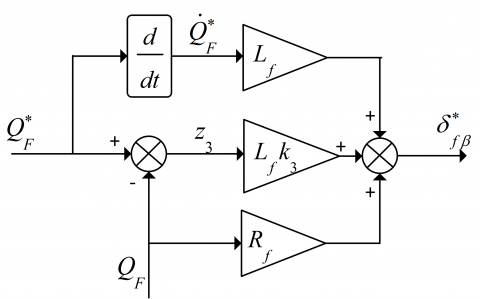Figure 5. Backstepping controller block diagram of the reactive power Once the intermediate voltage${\delta}^*_{f\beta}$is obtained, the reference voltage$v^*_{f\beta}$can be calculated using (7) as follows:$\mathrm { v } _ { \mathrm { f } \beta } ^ { * } = \frac { \mathrm { v } _ { \beta } } { \mathrm { v } _ { \alpha } ^ { 2 } + \mathrm { v } _ { \beta } ^ { 2 } } \delta _ { \mathrm { fex } } ^ { * } + \frac { \mathrm { v } _ { \alpha } } { \mathrm { v } _ { \alpha } ^ { 2 } + \mathrm { v } _ { \beta } ^ { 2 } } \delta _ { \mathrm { f } \beta } ^ { * } + \mathrm { v } _ { \beta }$(33) 4. DC-DC Boost Converter Modeling and Control 4.1 DC-DC boost converter modeling Figure. 6 represents the scheme of the DC-DC boost converter. The state space model for this converter is represented by the dynamic equations below:$\frac{dV_{PV}}{dt}=\frac{1}{C_{PV}}I_{PV}-\frac{1}{C_{PV}}I_{LPV}\frac{dI_{LPV}}{dt}=\frac{1}{L_{PV}}V_{PV}-\frac{1}{L_{PV}}(1-D)V_{dc}$(34) 4.2 Backstepping control of DC-DC boost converter The backstepping control approach is proposed again for the DC-DC boost converter to extract the maximum of power from the PV array. As shown in Figure. 6, two backstepping controllers are needed to control the photovoltaic generator output voltage and current. The control method of the voltage is fulfilled for the boost converter by controlling the voltage Vpv of the PV generator to its reference V*pv offered by perturb and observe (P&O) maximum power point tracking algorithm (MPPT). The output of the voltage loop controller and the PV current compensation provides the current reference I*Lpv of the inner loop current controller. So, the duty cycle of the converter is provided by the current control loop and the PV voltage Vpv and Vdv compensations. ## 6.pngFigure 6. Backstepping control of DC-DC boost converter The needed backstepping controllers are preformed based on a decomposition of the global model given by (34) into two subsystems, as follows: Subsystem 1:$\frac { \mathrm { d } \mathrm { V } _ { \mathrm { PV } } } { \mathrm { dt } } = \frac { 1 } { \mathrm { C } _ { \mathrm { PV } } } \mathrm { I } _ { \mathrm { PV } } - \frac { 1 } { \mathrm { C } _ { \mathrm { PV } } } \mathrm { I } _ { \mathrm { LPV } }$(35) In this first subsystem, the current ILPV is considered as a variable control while the PV output voltage VPV is considered as an output variable. Subsystem 2: In the second subsystem described by the equation (36), the duty cycle is considered as a variable control and the PV current ILPV as an output variable.$\frac { \mathrm { dI } _ { \mathrm { LPV } } } { \mathrm { dt } } = \frac { 1 } { \mathrm { L } _ { \mathrm { PV } } } V _ { \mathrm { PV } } - \frac { 1 } { \mathrm { L } _ { \mathrm { PV } } } ( 1 - \mathrm { D } ) \mathrm { V } _ { \mathrm { dc } }$(36) 4.2.1 PV voltage backstepping controller synthesis The synthesis of the desired backstepping regulator of the voltage VPV based on the first subsystem, defined by the equation (35), is analyzed as follows: The variable error zVpv is defined by:$Z_{Vpv}=V^*_{PV}-V_{PV}$(37) The dynamics of the error zVpv is given by:$\frac { \mathrm { d } \mathrm { Z } _ { \mathrm { Vp } v } } { \mathrm { dt } } = \frac { \mathrm { d } V _ { \mathrm { PV } } ^ { * } } { \mathrm { dt } } - \left( \frac { 1 } { \mathrm { C } _ { \mathrm { PV } } } \mathrm { I } _ { \mathrm { PV } } - \frac { 1 } { \mathrm { C } _ { \mathrm { PV } } } \mathrm { I } _ { \mathrm { LPV } } ^ { * } \right)$(38) The Lyapunov candidate function is chosen as:$V_{Vpv}=\frac{1}{2}z^2_{Vpv}$(39) The derivative of the function (39) is:$\frac { \mathrm { d } \mathrm { V } _ { \mathrm { Vpv } } } { \mathrm { dt } } = \mathrm { Z } _ { \mathrm { Vp } } \left( \frac { \mathrm { d } V _ { \mathrm { PV } } ^ { * } } { \mathrm { dt } } - \left( \frac { 1 } { \mathrm { C } _ { \mathrm { PV } } } \mathrm { I } _ { \mathrm { PV } } - \frac { 1 } { \mathrm { C } _ { \mathrm { PV } } } \mathrm { I } _ { \mathrm { LPV } } ^ { * } \right) \right)$(40) The stability of the system is guaranteed when the derivative of the Lyapunov function is negative; that can be realized by choosing the derivative of zVpv as:$\frac{dz_{Vpv}}{dt}=-k_{Vpv}z_{Vpv}$(41) with kVpv is a positive constant. Hence, the reference current$I^*_{LPV}$can be calculated as given in equation (42) and its controller block diagram is given by the Figure. 7.$\mathrm { I } _ { \mathrm { LPV } } ^ { * } = - \mathrm { I } _ { \mathrm { PV } } - \mathrm { k } _ { \mathrm { Vpv } } \mathrm { C } _ { \mathrm { PV } } \left( \mathrm { V } _ { \mathrm { PV } } ^ { * } - \mathrm { V } _ { \mathrm { PV } } \right) + \mathrm { C } _ { \mathrm { PV } } \frac { \mathrm { d } V _ { \mathrm { PV } } ^ { * } } { \mathrm { dt } }$(42) ## 7.png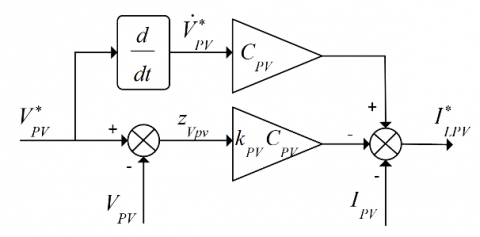Figure 7. Backstepping controller block diagram of the PV output voltage 4.2.2 PV current backstepping controller synthesis The synthesis of the desired backstepping controller of the current ILPV using the second subsystem defined by the equation (36) is analyzed as follows: The variable error zILpv is defined by:$Z_{ILpv}=I^*_{LPV}-I_{LPV}$(43) The dynamics of the error zILpv is given by:$\frac { \mathrm { d } \mathrm { z } _ { \mathrm { ILpv } } } { \mathrm { dt } } = \frac { \mathrm { d } \mathrm { I } _ { \mathrm { PV } } ^ { * } } { \mathrm { dt } } - \left( \frac { 1 } { \mathrm { L } _ { \mathrm { PV } } } \mathrm { V } _ { \mathrm { PV } } - \frac { 1 } { \mathrm { L } _ { \mathrm { PV } } } \left( 1 - \mathrm { D } ^ { * } \right) \mathrm { V } _ { \mathrm { dc } } \right)$(44) The Lyapunov candidate function is chosen as:$V_{ILpv}=\frac{1}{2}z^2_{ILpv}$(45) The derivative of the function (45) is:$\frac { \mathrm { d } \mathrm { V } _ { 2 } } { \mathrm { dt } } = \mathrm { z } _ { \mathrm { ILpv } } \left( \frac { \mathrm { d } \mathrm { I } _ { \mathrm { PV } } ^ { * } } { \mathrm { dt } } - \left( \frac { 1 } { \mathrm { L } _ { \mathrm { PV } } } \mathrm { V } _ { \mathrm { PV } } - \frac { 1 } { \mathrm { L } _ { \mathrm { PV } } } \left( 1 - \mathrm { D } ^ { * } \right) \mathrm { V } _ { \mathrm { dc } } \right) \right)$(46) The stability of the system is ensured by choosing the derivative of zILpv as:$\frac{dz_{ILpv}}{dt}=-k_{ILpv}z_{ILpv}$(47) with kILpv is a positive constant. Consequently, the reference of duty cycle D* can be calculated as given by the equation (48) and its controller block diagram is presented by the Figure 8:$\mathrm { D } ^ { * } = \frac { 1 } { \mathrm { V } _ { \mathrm { dc } } } \left( \mathrm { L } _ { \mathrm { PV } } \frac { \mathrm { d } \mathrm { I } _ { \mathrm { LPV } } ^ { * } } { \mathrm { dt } } - \mathrm { V } _ { \mathrm { PV } } + \mathrm { V } _ { \mathrm { dc } } + \mathrm { L } _ { \mathrm { PV } } \mathrm { k } _ { \mathrm { ILpv } } \left( \mathrm { I } _ { \mathrm { LPV } } ^ { * } - \mathrm { I } _ { \mathrm { LPV } } \right) \right)$(48) ## 8.pngFigure 8. Backstepping controller block diagram of the PV current 5. Principle of Prototyping Processor-In-The-Loop In PIL simulation, an embedded platform executing the control algorithm is connected to a host computer in which the physical system model is run. Then an assessment regarding the execution circumstances of the developed algorithm can be carried out aiming to optimize some important factors such as memory footprint, code size, and algorithm execution required time. The principle of PIL based development is depicted in Figure 9. ## 9.png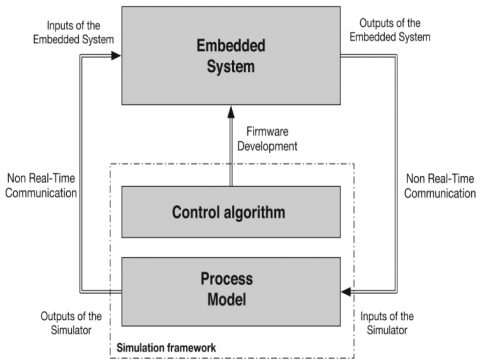Figure 9. Block diagram of embedded system connected to a PIL simulator The PIL prototyping permits verification and validation of the digital implementation of the control algorithms using a real DSP board, and this before embedding it into a real power environment. The interest of PIL prototyping is to validate the digital control algorithms implementation of the control part while simulating the power part by computer. Therefore, it is possible to validate the control algorithms in a virtual environment where the control algorithms could have corrected and modified without costly hardware iteration. This leads to decrease the cost of a project as well as the development time. Furthermore, it is possible to evaluate the performance of the system control algorithms and the weak points can be detected in this virtual environment while eliminating the risk of damaging all or part of the electrical system. In order to perform the PIL co-simulation, a communication link has to be set up between the host and target. In the given board of study, UART (Universal Asynchronous Receiver Transmitter) communication is used with standard communication protocol as defined by the manufacturer. The port parameter settings are fixed from PC as defined by manufacturer and the application programming interface (API). As STM DSP board has several serial communication options, a specific UART communication port can be set, which enable the embedded systems to send and receive the information to external devices. In addition, the Simulink model time domain and the real-time in the DSP hardware domain should be synchronized which is fixed in this study to$T_s=1\mu s$. Figure. 10(a) shows the connection between the serial communication Transmit Data (Tx) pin of the transmitter with Received Data (Rx) pin of the receiver. Note that the transmitter and receiver should have a common ground. In the given study, USB to RS232 TTL UART Prolific FTDI232 (serial communication) converter is used to communicate between the host PC and target board. The host, target device/board, and the communication link are shown in Figure. 10. ## 10.png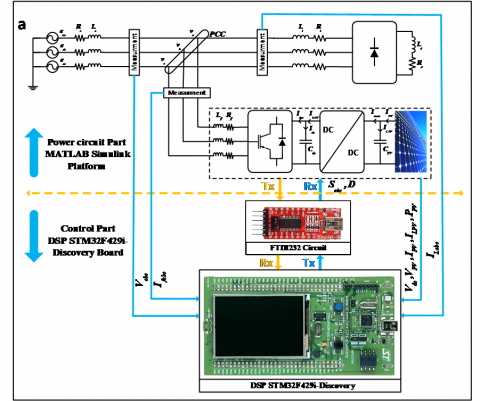## 10a.pngFigure 10. Processor-in-the-loop technique: (a) host device/board, and communication link, (b) PIL co-simulation platform 5.1 Co-simulation results In order to validate the proposed control method and assess its performances, the system has been modeled using embedded Matlab functions, and it is co-simulated through the processor-in-the-loop technique using STM32F429i-discovery DSP board under time step simulation$T_s=1\mu s\$ for both presented control techniques. The co-simulation parameters of the proposed PV-SAPF and its control as well as those of the PV generator are gathered in Tables 1 and 2, respectively. It is question also to exhibit the capability of the system to guarantee an active and reactive powers sharing between the shunt active power filter and distribution utility grid at the PCC under unexpected variations of load condition. Where at t=0.15 s another linear load is connected to PCC with the previous load, and is disconnected again at t=0.3 s. The system has examined at the standard test conditions: constant irradiation 1000 W/m² and a constant temperature 25°. The results of the system behavior for both backstepping and traditional PI controllers based direct power control are given in the Figures 12 to 16.

The source current before compensation and its harmonic spectrum are shown in Figure 11; the Figure 11(a) shows a substantial amount of harmonics in the source current with a total harmonic distortion (THD) equal to 28.96 % as can be seen in the Figure 11(b).

Table 1. PV-SAPF system parameters

 SAPF side RMS value of phase voltage 220 V DC-link capacitor Cdc 5 mF Source impedance Rs, Ls 1.6 mΩ ,100 mΩ Filter impedance Rf, Lf 1 mΩ , 350 μH Line impedance Rl, Ll 2.7 mΩ  , 25 μH Diode rectifier load Rd, Ld 5 mΩ  , 2.6 μH Fundamental frequency fs 50 H DC-link voltage reference V*dc 700 V Control constants parameters k1,k2=k3 170, 5x109 PV side system parameters Inductance LPV 5 mH Capacitance CPV 55 mF Control constants parameters kVpv,kILpv 13x106 , 5x103
Table 2. The array parameters of the (BP Solar’s SX 150 solar array) at the standard conditions
 Maximum power ( PMax) 150 W Voltage at PMax  ( Vmp ) 34.5 V Current at PMax ( Imp ) 4.35 A Warranted minimum PMax 140 W Short-circuit current ( Isc) 4.75 A Open-circuit voltage ( Voc ) 43.5 V Maximum system voltage 600 V Temperature coefficient of Isc (0.065 ±0.015) %/C° Temperature coefficient of Voc -(160 ±20)mV/C° Temperature coefficient of power -(0.5 ±0.05) %/C° NOCT 47 ±2 C°
The dynamic responses of the PV-SAPF system controlled by backstepping approach during a sudden load variation are illustrated in Figure 12. The waveforms include the injected filter current, three phase source currents, source voltage and current, source current harmonic spectrum, and DC-link capacitor voltage. In this test, another linear load with the same value as the first one is connected to the PCC at t=0.15s and it is disconnected again after 0.15s. From Figure. 12(b), the source current is sinusoidal and in phase with its corresponding voltage even during the step change caused by the added extra load as shown in the same Figure. The THD of the source current is reduced from 28.96 % before compensation to 1.55 % after compensation as shown in Figure 12(e). Consequently, the source current is almost free of harmonic reactive current components, which leads to a unity power factor operation. As indicated in Figure 12(d), the DC-link voltage across the capacitor is managed constant during the load change with a drop voltage lower than 30 V; the recovery time is about 0.025 s. Thus, this result confirms the efficiency of the DC-link voltage backstepping control.

Using traditional PI controller, the abovementioned PV-SAPF system waveforms under sudden load changes are shown in Figure 14. A unity power factor operation is achieved with approximately sinusoidal source current in phase with its corresponding voltage. Using this controller, the THD is reduced from 28.96 % to 2.28 % as shown in Figure 14(e). The DC-link voltage is maintained constant during the connection and disconnection of the extra load as illustrated in Figure. 14(d). Even though, the THD is low with an almost sinusoidal source current, the compensation effectiveness of the PI controller is still inferior compared to the proposed controller in terms of power quality improvement.

The waveforms of the corresponding exchanged active and reactive powers between load, grid, and shunt active power filter are shown in Figures 13, and 15, for both proposed and PI control methods, respectively. Figures 13(a) and 15(a) show that the active power of the shunt active power filter is added to the grid power with a view to supply the load active power demand. Furthermore, the reactive powers waveforms, shown in Figures 13(b) and 15 (b), argue that the shunt active power filter fulfills the load reactive power demand. This is demonstrated by a zero value of the grid reactive power. Figures 13(c) and 15(c) show the active power produced by the PV system, which is clearly equal to the maximum power of PV generator at the standard conditions. The time response of the generated PV active power is about 0.03 s for the PI control and about 0.005 s for the proposed backstepping control. The error between the generated PV power and its reference in steady state is about 200 W for the PI control and about 50 W for the proposed backstepping control. Accordingly, these results confirm again the efficiency of the DC-DC boost converter backstepping controller compared to the traditional PI controller.

Under continuous operation conditions, the values of SAPF inductors may vary. A small variation of these inductances is a reason not only of an incorrect extent of instantaneous active and reactive powers flow generated by the shunt active power filter but also high harmonic distortion. The effect of these parameters variation on the source current harmonic distortion is analyzed with different inductance values as indicated in Figure. 16. It is easily to find out that the THD decreases with the increase of the filter inductances. It is worth noticing that the proposed backstepping direct power control gives the best results for all same variations of inductors parameters.

The effect of simulation time step Ts is affects directly the THD for the proposed controller, if the time step Ts  is decreased, that makes the source current purely sinusoidal which leads to reduce the value of the THD. Otherwise, if the time step Ts  is increased then the source current won’t be purely sinusoidal and that increase its THD value. Hence, in this paper, we have chosen a very small value of Ts  equals to 1µs to get the better performances for the backstepping controller.

## 11a.png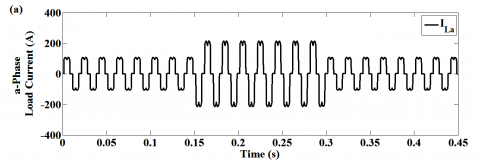## 11b.pngFigure 11. PV-SAPF system responses without filtering function: (a) supply current before harmonics compensation in steady state, (b) harmonic spectrum of supply current

## 12a.png## 12b.png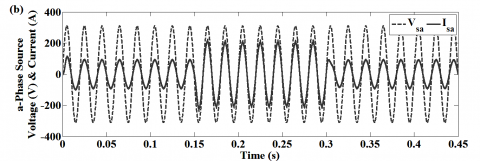## 12c.png## 12d.png## 12e.pngFigure 12. Dynamic responses of PV-SAPF system controlled by backstepping control: (a) filter current, (b) source voltage and source current of a-phase, (c) three-phase source currents, (d) DC-link voltage, (e) harmonic spectrum of line current

## 13a.png## 13b.png## 13c.pngFigure 13. Dynamic responses of PV-SAPF system controlled by backstepping control: (a) grid, load, and voltage source inverter active powers, (b) grid, load, and voltage source inverter reactive powers, (c) power generated by the PV generator (GPV), maximum power point of PVG (MPP)

## 14a.png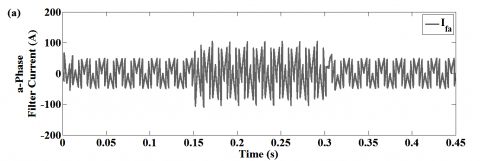## 14b.png## 14c.png## 14d.png## 14e.pngFigure 14. Dynamic responses of PV-SAPF system controlled by PI control: (a) filter current, (b) source voltage and source current of a-phase, (c) Three-phase source currents, (d) DC-link voltage, (e) Harmonic spectrum of line current

## 15a.png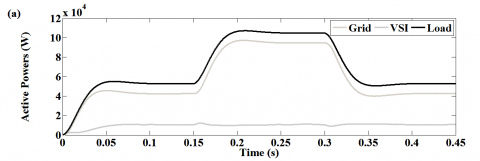## 15b.png## 15c.png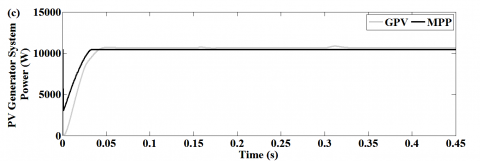Figure 15. Dynamic responses of PV-SAPF system controlled by PI control: (a) grid, load, and voltage source inverter active powers, (b) grid, load, and voltage source inverter reactive powers, (c) power generated by the PVG, MPP of PVG

## 16.jpgFigure 16. Comparative study between PI and backstepping direct power controls: line current THD versus SAPF inductor value

6. Conclusion

This paper has attained a direct power control based on a nonlinear backstepping approach for grid-connected PV system acting as a shunt active power filter. The proposed control scheme is designed to investigate the DC-link voltage regulation, harmonics elimination, reactive power compensation, and power flow sharing between PV generator and the utility grid. Co-simulation results based on processor-in-the-loop technique demonstrate that the backstepping based direct power control strategy can achieve not only the regulation of power factor observed at the PCC between the nonlinear load and the power distribution system but also manifest excellent transient responses during load variations. Furthermore, the DC-DC boost side backstepping control exhibits a good power flow sharing between the PV generator and the utility grid. When compared to the conventional PI control, the backstepping control displays substantial improvements in terms of supply current harmonic content and PV power sharing. These results assert that the backstepping control strategy offers higher performances than the conventional control.

References

 Lekouaghet, B., Boukabou, A., Lourci, N., Bedrine, K. (2018). Control of PV grid connected systems using MPC technique and different inverter configureuration models. Electric Power Systems Research, 154: 287-298. http://dx.doi.org/10.1016/j.epsr.2017.08.027

 Tareen, W.U., Mekhilef, S., Seyedmahmoudian, M., Horan, B. (2017). Active power filter (APF) for mitigation of power quality issues in grid integration of wind and photovoltaic energy conversion system. Renewable and Sustainable Energy Reviews, 70: 635-655. http://dx.doi.org/10.1016/j.rser.2016.11.091

 Abdul Kadir, A.F., Khatib, T., Elmenreich, W. (2014). Integrating photovoltaic systems in power system: Power quality impacts and optimal planning challenges. International Journal of Photoenergy, 2014. http://dx.doi.org/10.1155/2014/321826

 Ouchen, S., Abdeddaim, S., Betka, A., Menadi, A. (2016). Experimental validation of sliding mode-predictive direct power control of a grid connected photovoltaic system, feeding a nonlinear load. Solar Energy, 137: 328-336. http://dx.doi.org/10.1016/j.solener.2016.08.031

 Agarwal, R.K., Hussain, I., Singh, B., Chandra, A., Al-Haddad, K. (2016). Improved power quality of three-phase grid connected Solar Energy Conversion System under grid voltages distortion and imbalances. Industry Applications Society Annual Meeting, 2016, pp. 1-8. http://dx.doi.org/10.1109/IAS.2016.7731823

 Tuyen, N.D., Fujita, G. (2015). PV-active power filter combination supplies power to nonlinear load and compensates utility current. IEEE Power and Energy Technology Systems Journal, 2: 32-42. http://dx.doi.org/10.1109/JPETS.2015.2404355

 Srinath, S., Poongothai, M.S., Aruna, T. (2017). PV integrated shunt active filter for harmonic compensation. Energy Procedia, 117: 1134-1144. http://dx.doi.org/10.1016/j.egypro.2017.05.238

 Romero-Cadaval, E., Spagnuolo, G., Franquelo, L.G., Ramos-Paja, C.A., Suntio, T., Xiao, W.M. (2013). Grid-connected photovoltaic generation plants: Components and operation. IEEE Industrial Electronics Magazine, 7: 6-20. http://dx.doi.org/10.1109/MIE.2013.2264540

 Calleja, H., Jimenez, H. (2004). Performance of a grid connected PV system used as active filter. Energy Conversion and Management, 45: 2417-2428. http://dx.doi.org/10.1016/j.enconman.2003.11.017

 Afghoul, H., Chikouche, D., Krim, F., Babes, B., Beddar, A. (2016). Implementation of fractional-order integral-plus-proportional controller to enhance the power quality of an electrical grid. Electric Power Components and Systems, 44: 1018-1028. http://dx.doi.org/10.1080/15325008.2016.1147509

 Braiek, M.B., Fnaiech, F., Al-Haddad, K. (2005). Adaptive controller based on a feedback linearization technique applied to a three-phase shunt active power filter. Industrial Electronics Society, 2005. IECON 2005. 31st Annual Conference of IEEE, 6. http://dx.doi.org/10.1109/IECON.2005.1569037

 Komurcugil, H., Kukrer, O. (2006). A new control strategy for single-phase shunt active power filters using a Lyapunov function. IEEE Transactions on Industrial Electronics, 53: 305-312. http://dx.doi.org/10.1109/TIE.2005.862218

 Rahmani, S., Mendalek, N., Al-Haddad, K. (2010). Experimental design of a nonlinear control technique for three-phase shunt active power filter. IEEE Transactions on Industrial Electronics, 57: 3364-3375. http://dx.doi.org/10.1109/TIE.2009.2038945

 Habiba, B., Abdelhalim, T. (2017). Harmonic Compensation in Five Level NPC Active Filtering: Analysis, Dimensioning and Robust Control Using IT2 FLC. AMSE JOURNALS-AMSE IIETA publication-2017-Series: Advances C, 72(4): 227-247. http://dx.doi.org/10.18280/ama_c.720403

 Matas, J., De Vicuna, L.G., Miret, J., Guerrero, J.M., Castilla, M. (2008). Feedback linearization of a single-phase active power filter via sliding mode control. IEEE Transactions on Power Electronics, 23: 116-125. http://dx.doi.org/10.1109/TPEL.2007.911790

 Oucheriah, S., Guo, L. (2013). PWM-based adaptive sliding-mode control for boost DC–DC converters. IEEE Transactions on Industrial Electronics, 60: 3291-3294. http://dx.doi.org/10.1109/TIE.2012.2203769

 Salimi, M., Siami, S. (2015). Cascade nonlinear control of DC-DC buck/boost converter using exact feedback linearization. Electric Power and Energy Conversion Systems (EPECS), 2015 4th International Conference on, pp. 1-5. http://dx.doi.org/10.1109/EPECS.2015.7368525

 Chaoui, A., Gaubert, J.P., Krim, F. (2010). Power quality improvement using DPC controlled three-phase shunt active filter. Electric Power Systems Research, 80: 657-666. http://dx.doi.org/10.1016/j.epsr.2009.10.020

 Zhang, M., Lv, R., Hu, E., Xu, C., Yang, X. (2011). Research on direct power control strategy. Artificial Intelligence, Management Science and Electronic Commerce (AIMSEC), 2011 2nd International Conference on, pp. 7195-7197. http://dx.doi.org/10.1109/AIMSEC.2011.6010838

 Fouad, B., Ali, C., Samir, Z., Salah, S. (2017). Direct Torque Control of Induction Motor Fed by Three-level Inverter Using Fuzzy Logic. AMSE JOURNALS-AMSE IIETA publication-2017-Series: Advances C, 72(4): 248-265. http://dx.doi.org/10.18280/ama_c.720404

 Cichowlas, M., Malinowski, M., Kazmierkowski, M.P., Sobczuk, D.L., Rodríguez, P., Pou, J. (2005). Active filtering function of three-phase PWM boost rectifier under different line voltage conditions. IEEE transactions on industrial electronics, 52: 410-419. http://dx.doi.org/10.1109/TIE.2005.843915

 Guo, R., Huang, D., Zhang, L. (2005). Chaotic synchronization based on Lie derivative method. Chaos, Solitons & Fractals, 25: 1255-1259. http://dx.doi.org/10.1016/j.chaos.2004.11.067

 Byrnes, C., Isidori, A. (2000). Output regulation for nonlinear systems: an overview. International Journal of Robust and Nonlinear Control, 10: 323-337. http://dx.doi.org/10.1002/(SICI)1099-1239(20000430)10:5%3C323::AID-RNC483%3E3.0.CO;2-G

 Watanabe, E.H., Akagi, H., Aredes, M. (2008). Instantaneous pq power theory for compensating nonsinusoidal systems, in Nonsinusoidal Currents and Compensation, 2008. ISNCC 2008. International School on, pp. 1-10. http://dx.doi.org/10.1109/ISNCC.2008.4627480

 Bouzidi, M., Benaissa, A., Barkat, S., Bouafia, S., Bouzidi, A. (2017). Virtual flux direct power control of the three-level NPC shunt active power filter based on backstepping control. International Journal of System Assurance Engineering and Management, 8: 287-300. http://dx.doi.org/10.1007/s13198-016-0433-3

 Zhang, Y., Fidan, B., Ioannou, P.A. (2003). Backstepping control of linear time-varying systems with known and unknown parameters. IEEE Transactions on Automatic Control, 48: 1908-1925. http://dx.doi.org/10.1109/TAC.2003.819074

 Layadi, N., Zeghlache, S., Benslimane, T., Berrabah, F. (2017). Comparative analysis between the rotor flux oriented control and backstepping control of a double star induction machine (DSIM) under open-phase fault. AMSE JOURNALS-AMSE IIETA publication-2017-Series: Advances C, 72(4): 292-311. http://dx.doi.org/10.18280/ama_c.720407